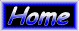Root Mean Chord by Quadratic Formula by Michael A. Morrow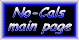Calculating the Root Mean Chord Using the Quadratic Equation

The Root Mean Chord is the chord on the wing where the area of the wing to the left of the Root Mean Chord is equal to the area to the right of the Root Mean Chord.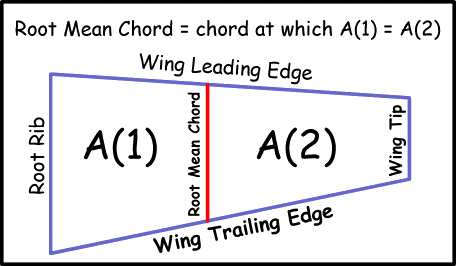Using the Quadratic Formula to calculate the location of the root mean chord will give two answers, one of which will be complete nonsense, and the other of which will locate the distance of the Root Mean Chord from the wing root. In order to use the formula, the variables will have to be defined. Here is a drawing of a wing half showing the variables and where they are measured.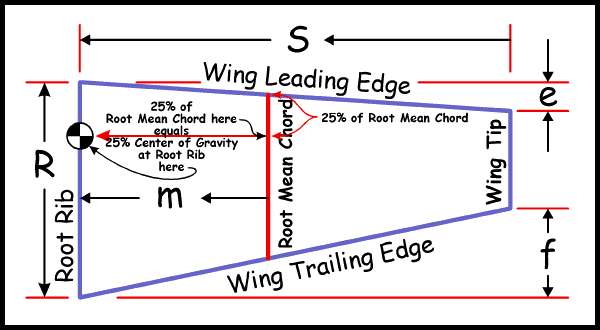. . . where the variables are defined as:

S = span of the wing segment
you are calculating the Root Mean Chord for

R = root chord of the wing segment

e = sweep back of wing leading edge measured at the wing tip

f = sweep forward of wing trailing edge measured at the wing tip

Area of wing half = A = (S*R - (S*e)/2) - (S*f)/2

M = distance from wing root to Root Mean Chord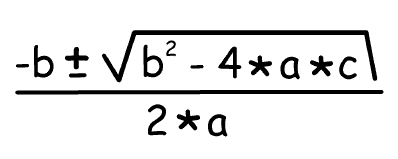Where: a = e + f b = (2 * R * S) c = S * A
1) On a plan of the wing, measure the values S, R, e, and F.

2) Using the values S, R, e, and f, calculate a, b, and c, using the formulas already given:
a = e + f

b = (2 * R * S)

c = S * A

The quadratic formula has two forms:

Minus 'b' plus the square root: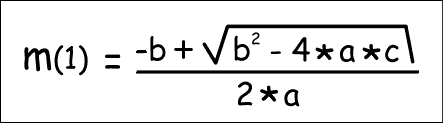and minus 'b' MINUS the square root: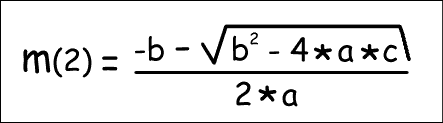3) Plug the values you've calculated for a, b, and c into each of the above quadratic formulas, and solve for the answers m(1) and m(2).

One value of 'm' will clearly be nonsense (probably bigger than the span of the wing segment).

The other value of 'm' will be between the root rib and the tip rib, and will be the distance from the Root Rib to the Root Mean Chord: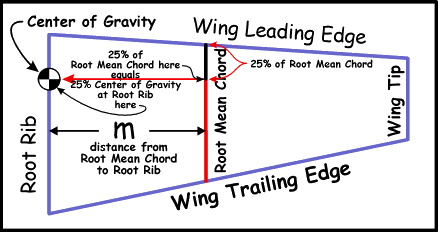4) With value of 'm', the distance from the Root Rib to the Root Mean Chord you've just calculated, draw the Root Mean Chord on the wing plan.

5) Measure the length of the Root Mean Chord, and divide by 4. This will be your 25% center of gravity on the Root Mean Chord.

6) Using the length calculated in Step 5, measure that length back from the leading edge on the Root Mean Chord, and mark that spot.

7) Draw a horizontal line straight across from the point marked in Step 6 to the Root Rib. This will be your 25% center of gravity at the wing root. This is where you will balance the model.

Congratulations! You've just calculated a Center of Gravity using the Quadratic Formula method!

Now, back to your flight-trimming process in progress!

 Back to the Flight Trimming a No-Cal pageMichael A. Morrow's AERO ACES - Doing Business since 1995 ! AERO ACES is a TRADEMARK of Michael A. Morrow. © All Images, drawings, plans, articles, and kits, are Registered & Copyrighted by Michael A. Morrow